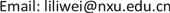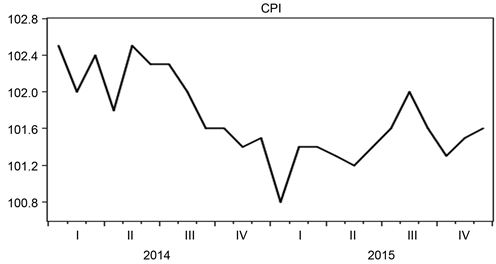﻿ 模糊回归组合模型在CPI预测中的应用 Fuzzy Regression Combination Model and Its Application in CPI Forecasting

Operations Research and Fuzziology
Vol.08 No.01(2018), Article ID:23828,9 pages
10.12677/ORF.2018.81004

Fuzzy Regression Combination Model and Its Application in CPI Forecasting

Lili Wei, He Li

School of Mathematics and Statistics, Ningxia University, Yinchuan NingxiaReceived: Feb. 6th, 2018; accepted: Feb. 19th, 2018; published: Feb. 26th, 2018ABSTRACT

A fuzzy regression combination forecasting method is proposed and applied to the CPI forecasting in this paper. The method can synthesize the advantages of fuzzy regression and combination forecasting, and give a practical predicted interval value of CPI. Firstly, the quadratic curve model and the time series model be selected as the single prediction model, and the prediction results are fuzzified. Secondly, the fuzzy regression combination model is established by minimizing the fuzzy degree for the given goodness-of-fit to solve the optimal weight coefficient. Finally, the CPI forecasting results show that the fuzzy regression model is better than the single prediction model, which can improve the prediction accuracy of CPI and is suitable for short-term prediction of CPI.

Keywords:Fuzzy Regression, Combination Forecasting, CPI1. 引言

CPI是进行国民经济核算的重要指标。一般来说，国家宏观经济调控政策的出台力度受CPI的直接影响。对CPI进行定量预测研究，有利于我们认清当下经济发展的趋势，以及在制定经济政策时可以提供可靠的依据和建议。本文提出了一种模糊回归组合模型，对我国近年CPI数据进行短期的预测研究。该方法能综合模糊回归和组合预测的优点，给出更符合实际的CPI预测区间值。首先，分别选取二次曲线模型和时间序列模型作为单项预测模型对CPI进行预测，并将单项预测结果进行模糊化。其次，在给定拟合度的条件下，通过极小化模糊度来建立模糊回归组合模型。最后，应用计算机软件求得最优权重系数。

2. 模糊回归理论

2.1. 模糊集与模糊数

1) 当且仅当 $A$ 为经典集合时， $d\left(A\right)=0$

2) $\forall x\in X$ ，当且仅当 $A\left(x\right)\equiv 0.5$ 时， $d\left(A\right)=1$

3) $\forall x\in X$ ，当 $B\left(x\right)\le A\left(x\right)\le 0.5$ 时， $d\left(B\right)\le d\left(A\right)$

4) $A\in \mathcal{F}\left(X\right)$$d\left(A\right)=d\left({A}^{c}\right)$

$J\left(A\right)={\int }_{-\infty }^{\infty }|A\left(x\right)-{A}_{0.5}\left(x\right)|\text{d}x$

$h\left(A,B\right)=\underset{x\in X}{\vee }\left(A\left(x\right)\wedge B\left(x\right)\right)$

$A\left(x\right)=\left\{\begin{array}{ll}\left(x-a\right)/\left(b-a\right),\hfill & a\le x\le b,\hfill \\ \left(x-c\right)/\left(b-c\right),\hfill & b\le x\le c,\hfill \\ 0,\hfill & \text{\hspace{0.17em}}\text{\hspace{0.17em}}\text{\hspace{0.17em}}其他,\hfill \end{array}$

$A\left(x\right)=\left\{\begin{array}{ll}1-|x-c|/\sigma ,\hfill & c-\sigma \le x\le c+\sigma ,\hfill \\ 0,\hfill & \text{\hspace{0.17em}}\text{\hspace{0.17em}}\text{\hspace{0.17em}}\text{\hspace{0.17em}}\text{\hspace{0.17em}}\text{\hspace{0.17em}}\text{\hspace{0.17em}}\text{\hspace{0.17em}}其他.\hfill \end{array}$

1) $tA=\left(tc,t\sigma \right)\text{\hspace{0.17em}}\left(t>0\right)$ ; 2) $A+B=\left(c+d,\sigma +\delta \right)$

2.2. 模糊线性回归模型

$Y={A}_{1}{x}_{1}+{A}_{2}{x}_{2}+\cdots +{A}_{k}{x}_{k}$(1)

${A}_{j}\left(z\right)=\left\{\begin{array}{ll}1-\left(z-{c}_{j}\right)/{\sigma }_{j},\hfill & |z-{c}_{j}|\le {\sigma }_{j},\hfill \\ 0,\hfill & \text{\hspace{0.17em}}\text{\hspace{0.17em}}\text{\hspace{0.17em}}\text{\hspace{0.17em}}\text{\hspace{0.17em}}\text{\hspace{0.17em}}其他.\hfill \end{array}$

$z$ 是模糊回归系数的精确值， ${c}_{j}$${\sigma }_{j}$ 分别是模糊回归系数的中心和扩展。

${Y}_{i}=\left({\sum }_{j=1}^{k}{c}_{j}{x}_{ji},{\sum }_{j=1}^{k}{\sigma }_{j}|{x}_{ji}|\right)\text{\hspace{0.17em}}\left(i=1,2,\cdots ,n\right)$

1) 对于给定参数 $H,0\le H\le 1$ ，使得 ${Y}_{i}\left({y}_{i}\right)\ge H$

2) 由于对称三角形模糊数的模糊度与扩展 ${\sigma }_{i}$ 有关。因而定义模型(1)的糊度为

$J={\sigma }_{1}+{\sigma }_{2}+\cdots +{\sigma }_{k}$

$\begin{array}{l}\mathrm{min}J={\sum }_{j=1}^{k}{\sigma }_{j}\\ \text{s}\text{.t}\text{.}{y}_{i}\le {\sum }_{j=1}^{k}{c}_{j}{x}_{ji}+\left(1-H\right){\sum }_{j=1}^{k}{\sigma }_{j}|{x}_{ji}|,\\ \text{}{y}_{i}\ge {\sum }_{j=1}^{k}{c}_{j}{x}_{ji}-\left(1-H\right){\sum }_{j=1}^{k}{\sigma }_{j}|{x}_{ji}|\text{,}\\ \text{ }\text{\hspace{0.17em}}\text{\hspace{0.17em}}\text{\hspace{0.17em}}i=1,2,\cdots ,n.\end{array}$ (2)

3. 模糊回归组合预测

$m$ 种单一模型对同一对象做预测，可构建的组合预测模型为

${f}_{t}={\omega }_{1}{f}_{1t}+{\omega }_{2}{f}_{2t}+\cdots +{\omega }_{m}{f}_{mt}={\sum }_{i=1}^{m}{\omega }_{i}{f}_{it}$(3)

$t$ 期的实际观测值 ${y}_{t}$ 模糊化描述为 ${\stackrel{˜}{y}}_{t}=\left({y}_{t},{\epsilon }_{t}\right)$${\epsilon }_{t}\left({\epsilon }_{t}\ge 0\right)$ 为给定的置信值。将 $t$ 期第 $i$ 种单项模型的预测值 ${f}_{it}$ 模糊化描述为 ${\stackrel{˜}{f}}_{it}=\left({f}_{it},{\sigma }_{i}\right)$$i=1,2,\cdots ,m$ ，其中 ${\sigma }_{i}$ 是置信值，取值与 ${f}_{it}$ 和置信水平有关，

${\sigma }_{i}=1.96\sqrt{{\sum }_{t=1}^{N}{\left({y}_{t}-{f}_{it}\right)}^{2}/\left(N-m-1\right)}$

${\stackrel{˜}{f}}_{t}={\omega }_{1}{\stackrel{˜}{f}}_{1t}+{\omega }_{2}{\stackrel{˜}{f}}_{2t}+\cdots +{\omega }_{m}{\stackrel{˜}{f}}_{mt}={\sum }_{i=1}^{m}{\omega }_{i}{\stackrel{˜}{f}}_{it}$(4)

${\stackrel{˜}{f}}_{t}$ 的隶属函数表示为

${\stackrel{˜}{f}}_{t}$${\stackrel{˜}{y}}_{t}$ 的拟合度表示为

${h}_{t}\left({\stackrel{˜}{f}}_{t},{\stackrel{˜}{y}}_{t}\right)=1-\frac{|{y}_{t}-{\sum }_{i=1}^{m}{\omega }_{i}{f}_{it}|}{{\sum }_{i=1}^{m}{\omega }_{i}{\sigma }_{i}+{\epsilon }_{t}}$

$J={\omega }_{1}{\sigma }_{1}+{\omega }_{2}{\sigma }_{2}+\cdots +{\omega }_{m}{\sigma }_{m}={\sum }_{i=1}^{m}{\omega }_{i}{\sigma }_{i}$

$\begin{array}{l}\mathrm{min}\text{}J={\sum }_{i=1}^{m}{\omega }_{i}{\sigma }_{i}\\ \text{s}\text{.t}\text{.}{h}_{t}\left({\stackrel{˜}{f}}_{t},{\stackrel{˜}{y}}_{t}\right)=1-\frac{|{y}_{t}-{\sum }_{i=1}^{m}{\omega }_{i}{f}_{it}|}{{\sum }_{i=1}^{m}{\omega }_{i}{\sigma }_{i}+{\epsilon }_{t}}\ge H,\\ \text{}{\sum }_{i=1}^{m}{\omega }_{i}=1,\text{\hspace{0.17em}}{\omega }_{i}\ge 0\text{\hspace{0.17em}}\left(i=1,2,\cdots ,m\right).\end{array}$ (5)

$\begin{array}{l}{e}_{t}^{+}=\mathrm{max}\left(\left({y}_{t}-{\sum }_{i=1}^{m}{\omega }_{i}{f}_{it}\right),0\right)=\left({y}_{t}-{\sum }_{i=1}^{m}{\omega }_{i}{f}_{it}\right)\vee 0,\\ {e}_{t}^{-}=\mathrm{min}\left(\left({y}_{t}-{\sum }_{i=1}^{m}{\omega }_{i}{f}_{it}\right),0\right)=\left({y}_{t}-{\sum }_{i=1}^{m}{\omega }_{i}{f}_{it}\right)\wedge 0.\end{array}$

$\begin{array}{l}\mathrm{min}\text{}J={\sum }_{i=1}^{m}{\omega }_{i}{\sigma }_{i},\\ \text{s}\text{.t}\text{.}{y}_{t}-{\sum }_{i=1}^{m}{\omega }_{i}{f}_{it}\le \left(1-H\right){\sum }_{i=1}^{m}{\omega }_{i}{\sigma }_{i}+\left(1-H\right){\epsilon }_{t},\\ \text{}{y}_{t}-{\sum }_{i=1}^{m}{\omega }_{i}{f}_{it}\ge \left(H-1\right){\sum }_{i=1}^{m}{\omega }_{i}{\sigma }_{i}-\left(1-H\right){\epsilon }_{t},\\ \text{}{\sum }_{i=1}^{m}{\omega }_{i}=1,{\omega }_{i}\ge 0\text{\hspace{0.17em}}\left(i=1,2,\cdots ,m\right).\end{array}$ (6)

4. CPI实例应用

1) 二次曲线预测模型 ${f}_{1t}$Figure 1. CPI trends

${f}_{1t}=102.7050-0.153805t+0.004535{t}^{2}$ (7)

2) 时间序列模型 ${f}_{2t}$

$\left\{\begin{array}{l}{y}_{t}={\beta }_{0}+{\beta }_{1}t+{\beta }_{2}{t}^{2}+\cdots +{\beta }_{k}{t}^{k}+{\mu }_{t},\hfill \\ \varphi \left(B\right){\mu }_{t}=\theta \left(B\right){\epsilon }_{t},\hfill \end{array}$

$\left\{\begin{array}{l}{y}_{t}=102.7-0.15t+0.004{t}^{2}+{\mu }_{t}\hfill \\ 0.7591{\mu }_{t}=0.5804{\epsilon }_{t}\hfill \end{array}$ (8)Table 1. Prediction results and errors of individual models

Fuzzy Regression Combination Model and Its Application in CPI Forecasting[J]. 运筹与模糊学, 2018, 08(01): 30-38. http://dx.doi.org/10.12677/ORF.2018.81004

1. 1. 陈世全, 郭嗣宗. 模糊预测[M]. 贵阳: 贵阳科技出版社, 1994.

2. 2. Tanaka, H., Uejima, S. and Asai, K. (1982) Linear Regression Analysis with Fuzzy Model. IEEE Transactions on Systems, Man and Cybernetics, 12, 903-907. https://doi.org/10.1109/TSMC.1982.4308925

3. 3. 吴冲, 潘启树, 李汉玲. 模糊线性回归预测[J]. 西安交通大学学报, 2000, 34(9): 100-102.

4. 4. 丁世飞, 陈述汉, 苏本堂. 多元模糊回归预测模型及应用[J]. 模糊系统与数学, 2000, 14(3): 94-98.

5. 5. Bates, J.M. and Granger, C.W.J. (1969) The Combination of Forecasts. Operational Research Society, 20, 451-468. https://doi.org/10.1057/jors.1969.103

6. 6. 吴祈宗, 宋颖. 一种模糊组合预测方法[J]. 北京理工大学学报, 2004, 24(4): 373-376.

7. 7. Zadeh, L.A. (1965) Fuzzy Sets. Information and Control, 8, 338-353. https://doi.org/10.1016/S0019-9958(65)90241-X

8. 8. 杨纶标, 高英仪. 模糊数学原理及应用[M]. 第五版. 广州: 华南理工大学出版社, 2011.